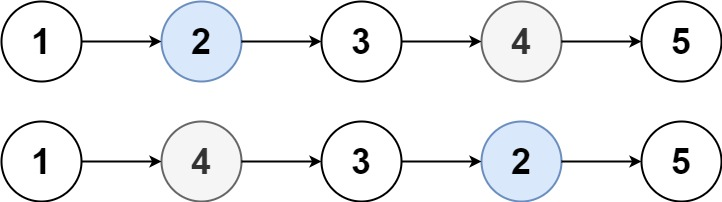# Solved Leetcode 1721: Swapping Nodes in a Linked List

### Swapping Nodes in a Linked List

You are given the head of a linked list, and an integer k.

Return the head of the linked list after swapping the values of the kth node from the beginning and the kth node from the end (the list is 1-indexed).

Example 1:Input: head = [1,2,3,4,5], k = 2
Output: [1,4,3,2,5]

Example 2:
Input: head = [7,9,6,6,7,8,3,0,9,5], k = 5
Output: [7,9,6,6,8,7,3,0,9,5]

Constraints:

• The number of nodes in the list is n.
• 1 <= k <= n <= 105
• 0 <= Node.val <= 100

Time Complexity
O(n), since we are traversing the entire linked list

Space Complexity
O(1)

Rate this post